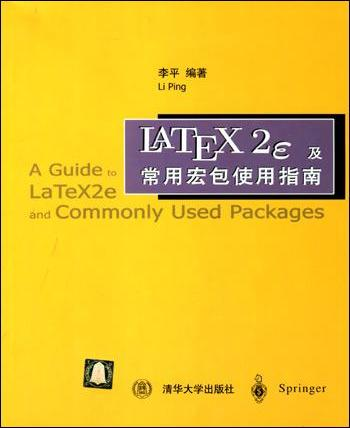CodeCogs 网站的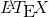服务接口格式如下所示：

http://latex.codecogs.com/svg.latex?LaTeX公式代码

\frac{1}{\pi}=\frac{2\sqrt{2}}{9801}\sum_{k=0}^\infty\frac{(4k)!(1103+26390k)}{(k!)^4396^{4k}}

1. \pi 表示希腊字母 π，\infty 表示 ∞。更多的符号请参见：Special Symbols
2. \frac{分子}{分母} 表示分数。另外，\tfrac{分子}{分母} 表示小号的分数。
3. \sqrt{被开方数} 表示平方根。另外，\sqrt[n]{x} 表示 n 次方根。
4. \sum_{下标}^{上标} 表示求和符号。另外，\prod 表示乘积符号，\int 表示积分符号。
5. _{下标} 和 ^{上标} 可以用在任何地方。如果上下标只是一个字符，可以省略 { 和 } 。
6. 此外，\ldots 和 \cdots 都表示省略号，前者排在基线上，后者排在中间。
7. 还有：\pm：±、\times：×、\div：÷ 。

## 长度中经常要用到长度的概念。最简单的长度就是一个十进制数（可带小数点，可正可负）加上一个长度单位。下面列出常用的一些长度单位。

• mm 毫米• cm 厘米 = 10 毫米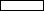• in 英寸 = 25.4 毫米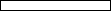• pt 点 = 1/72.27 英寸• bp 大点 = 1/72 英寸• pc pico = 12 点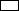• em 相当于当前字体的大写字母 M 的宽度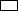• ex 相当于当前字体的小写字母 x 的高度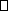中可以用 \hspace{length} 命令来改变水平方向的空白距离，其中 length 是空白距离的长度。例如 \hspace{1.5em} 。

## 定界符的尺寸

• 在左定界符的前面加上命令 \left，在右定界符的前面加上 \right 。
• 使用命令 \big，\Big，\bigg 和 \Bigg 。这些命令用于定界符的前面，将定界符尺寸逐渐增大。
• 另外，这几条命令还有变体，如 \bigl 和 \bigr 分别用于左定界符和右定界符的前面。